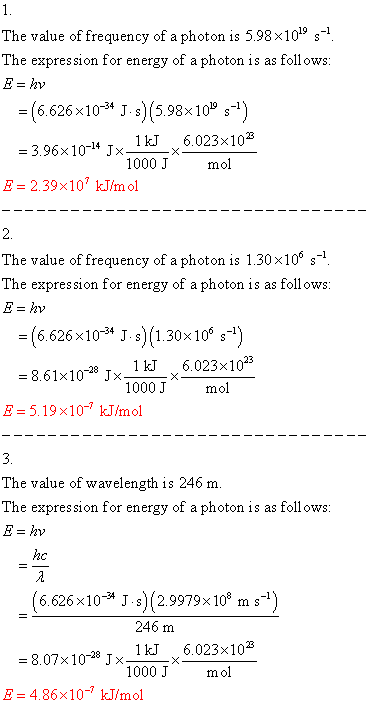# What is the energy of each of the following photons in kilojoules per mole?

What is the energy of each of the following photons in
kilojoules per mole? 1. 5.98×10195.98×1019 19 2. 1.30×1061.30×106 6 3.
= 246= 246 246

The value of frequency of a photon is 5.98×1019 s The expression for energy of a photon is as follows = (6.626×10-34 J. s) (5.98x 1019 s-1 41kJ6.023×103 -3.96×104 Jx 1KJx 1000 J mol E- 2.39×10 kJ/mol 2. The value of frequency of a photon is 1.30×10 s The expression for energy of a photon is as follows = (6.626×10-34 J s) (1.30×106 s-1) 8.61×10 Jx_1 kJ 6.023×103 1000 J mol E-5.19 x10-7 kJ/mol The value of wavelength is 246 m The expression for energy of a photon is as follows 246 m 21kJ 6.023×103 -8.07 x 10-28 J x-TKI-X 1000 J mol E-4.86×10- kJ/mol